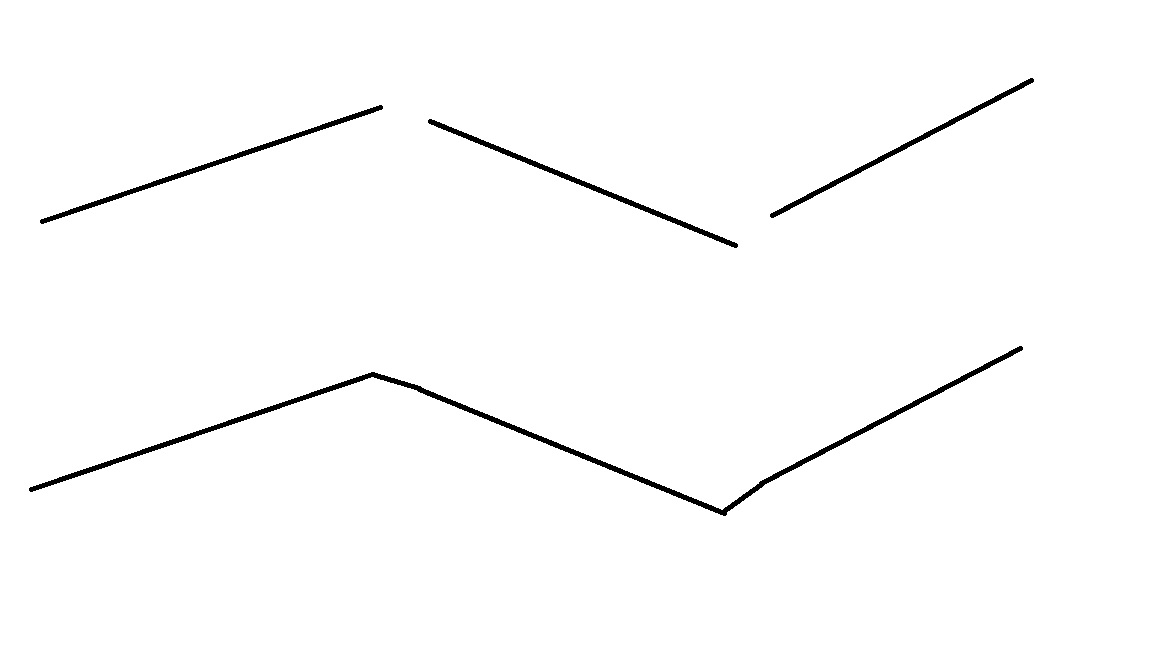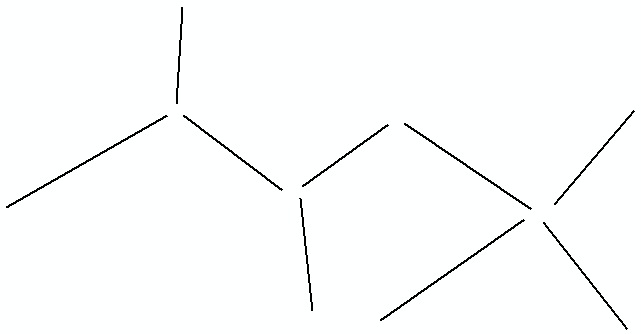# Connecting Discontinuous Line Segments

5439
3
01-28-2015 01:52 PMNew Contributor

I have a shape file with a series of line segments that are discontinuous, I want to join them together so they form a continuous line based on a common attribute from the attribute table. I already understand how to merge the lines using Merge in the editing tool bar, but I'm not clear on if there is a way to get those segments to link up as shown in the image below (I have the broken lines on top, I want the continuous line below) without manually snapping them together point by point, as it is a large file with numerous such segments. Is there a tool in ArcGIS that can do this? Thanks.  (Note: I've seen some other postings mention the Dissolve tool, but I don't see how it applies here).1 Solution

Accepted SolutionsbyEsri Esteemed Contributor

Hi Daniel Walters‌,

I saw your question and thought, let's give it a try...

Based on this input:Is creates this output:Using this code:

```import math

def main():
import arcpy

fc_in = r"D:\Xander\GeoNet\Nueva carpeta\test.gdb\lines"
fc_out = r"D:\Xander\GeoNet\Nueva carpeta\test.gdb\lines_out"
tolerance = 150 # max distance to snap to a node

# create dicts
dct_lines = getPolylineDict(fc_in)
dct_from, dct_to = getFromAndToNodes(dct_lines)

# loop through polylines
for oid, polyline in dct_lines.items():
# search candidates from point
pnt_from = dct_from[oid]
dist_min = tolerance + 0.01
pnt_found = None
for oid_from, pnt in dct_from.items():
if oid_from != oid:
dist = calcDistance(pnt_from, pnt)
if dist < dist_min:
dist_min = dist
pnt_found = arcpy.Point(pnt.X, pnt.Y)

for oid_to, pnt in dct_to.items():
if oid_to != oid:
dist = calcDistance(pnt_from, pnt)
if dist < dist_min:
dist_min = dist
pnt_found = arcpy.Point(pnt.X, pnt.Y)

# change line from point
if pnt_found != None:
polyline = insertPointAtStart(polyline, pnt_found)
dct_lines[oid] = polyline
dct_from, dct_to = getFromAndToNodes(dct_lines)

# search candidates to points
pnt_to = dct_to[oid]
dist_min = tolerance + 0.01
pnt_found = None
for oid_from, pnt in dct_from.items():
if oid_from != oid:
dist = calcDistance(pnt_to, pnt)
if dist < dist_min:
dist_min = dist
pnt_found = arcpy.Point(pnt.X, pnt.Y)

for oid_to, pnt in dct_to.items():
if oid_to != oid:
dist = calcDistance(pnt_to, pnt)
if dist < dist_min:
dist_min = dist
pnt_found = arcpy.Point(pnt.X, pnt.Y)

# change line end point
if pnt_found != None:
polyline = addPointAtEnd(polyline, pnt_found)
dct_lines[oid] = polyline
dct_from, dct_to = getFromAndToNodes(dct_lines)

# create list of polylines
lst_polylines = dct_lines.values()
arcpy.CopyFeatures_management(lst_polylines, fc_out)

def getPolylineDict(fc_in):
dct = {}
flds = ("OID@", "SHAPE@")
with arcpy.da.SearchCursor(fc_in, flds) as curs:
for row in curs:
dct[row] = row
del row, curs
return dct

def getFromAndToNodes(dct):
dct_from = {}
dct_to = {}
for oid, polyline in dct.items():
dct_from[oid] = polyline.firstPoint
dct_to[oid] = polyline.lastPoint
return dct_from, dct_to

def insertPointAtStart(polyline, pnt_found):
sr = polyline.spatialReference
lst_pnts = [pnt_found]
for part in polyline:
for pnt in part:
lst_pnts.append(pnt)
return arcpy.Polyline(arcpy.Array(lst_pnts), sr)

sr = polyline.spatialReference
lst_pnts = []
for part in polyline:
for pnt in part:
lst_pnts.append(pnt)
lst_pnts.append(pnt_found)
return arcpy.Polyline(arcpy.Array(lst_pnts), sr)

def calcDistance(pnt_to, pnt):
return math.hypot(pnt_to.X - pnt.X, pnt_to.Y - pnt.Y)

if __name__ == '__main__':
main()```

If you have any questions, just let me know...

Kind regards, Xander

3 RepliesbyEsri Esteemed Contributor

Hi Daniel Walters‌,

I saw your question and thought, let's give it a try...

Based on this input:Is creates this output:Using this code:

```import math

def main():
import arcpy

fc_in = r"D:\Xander\GeoNet\Nueva carpeta\test.gdb\lines"
fc_out = r"D:\Xander\GeoNet\Nueva carpeta\test.gdb\lines_out"
tolerance = 150 # max distance to snap to a node

# create dicts
dct_lines = getPolylineDict(fc_in)
dct_from, dct_to = getFromAndToNodes(dct_lines)

# loop through polylines
for oid, polyline in dct_lines.items():
# search candidates from point
pnt_from = dct_from[oid]
dist_min = tolerance + 0.01
pnt_found = None
for oid_from, pnt in dct_from.items():
if oid_from != oid:
dist = calcDistance(pnt_from, pnt)
if dist < dist_min:
dist_min = dist
pnt_found = arcpy.Point(pnt.X, pnt.Y)

for oid_to, pnt in dct_to.items():
if oid_to != oid:
dist = calcDistance(pnt_from, pnt)
if dist < dist_min:
dist_min = dist
pnt_found = arcpy.Point(pnt.X, pnt.Y)

# change line from point
if pnt_found != None:
polyline = insertPointAtStart(polyline, pnt_found)
dct_lines[oid] = polyline
dct_from, dct_to = getFromAndToNodes(dct_lines)

# search candidates to points
pnt_to = dct_to[oid]
dist_min = tolerance + 0.01
pnt_found = None
for oid_from, pnt in dct_from.items():
if oid_from != oid:
dist = calcDistance(pnt_to, pnt)
if dist < dist_min:
dist_min = dist
pnt_found = arcpy.Point(pnt.X, pnt.Y)

for oid_to, pnt in dct_to.items():
if oid_to != oid:
dist = calcDistance(pnt_to, pnt)
if dist < dist_min:
dist_min = dist
pnt_found = arcpy.Point(pnt.X, pnt.Y)

# change line end point
if pnt_found != None:
polyline = addPointAtEnd(polyline, pnt_found)
dct_lines[oid] = polyline
dct_from, dct_to = getFromAndToNodes(dct_lines)

# create list of polylines
lst_polylines = dct_lines.values()
arcpy.CopyFeatures_management(lst_polylines, fc_out)

def getPolylineDict(fc_in):
dct = {}
flds = ("OID@", "SHAPE@")
with arcpy.da.SearchCursor(fc_in, flds) as curs:
for row in curs:
dct[row] = row
del row, curs
return dct

def getFromAndToNodes(dct):
dct_from = {}
dct_to = {}
for oid, polyline in dct.items():
dct_from[oid] = polyline.firstPoint
dct_to[oid] = polyline.lastPoint
return dct_from, dct_to

def insertPointAtStart(polyline, pnt_found):
sr = polyline.spatialReference
lst_pnts = [pnt_found]
for part in polyline:
for pnt in part:
lst_pnts.append(pnt)
return arcpy.Polyline(arcpy.Array(lst_pnts), sr)

sr = polyline.spatialReference
lst_pnts = []
for part in polyline:
for pnt in part:
lst_pnts.append(pnt)
lst_pnts.append(pnt_found)
return arcpy.Polyline(arcpy.Array(lst_pnts), sr)

def calcDistance(pnt_to, pnt):
return math.hypot(pnt_to.X - pnt.X, pnt_to.Y - pnt.Y)

if __name__ == '__main__':
main()```

If you have any questions, just let me know...

Kind regards, XanderNew Contributor

Hi XanderBarkker,

Thanks a lot for your python script. It helped me a lot with a project am working on. I am running the script on a file and currently having some error. Please see below:Any help or input is welcomes. Thanks!Regular Contributor

nice job with python coding by Xander,

Also, nice to use the simple generalization tool called 'Extend Line' at ArcGIS Help (10.2, 10.2.1, and 10.2.2)

The following simple standalone is copied from the Help

`# Name: ExtendLine.py# Description:  Clean up street centerlines that were digitized without #                      having set proper snapping environments# import system modules import arcpyfrom arcpy import env# Set environment settingsenv.workspace = "C:/data"# Make backup copy of streets feature class, since modification with #  the Editing tools below is permanentstreets = "majorrds.shp"streetsBackup = "C:/output/Output.gdb/streetsBackup"arcpy.CopyFeatures_management(streets, streetsBackup)# Trim street lines to clean up danglesarcpy.TrimLine_edit(streets, "10 Feet", "KEEP_SHORT")# Extend street lines to clean up danglesarcpy.ExtendLine_edit(streets, "15 Feet", "EXTENSION")`